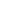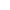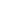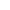# Module 06: Equating Methods in Classical Test Theory

• Learner - Free!

This instructional module is intended to promote a conceptual understanding of test form equating using traditional methods. The purpose of equating and the context in which equating occurs are described. The process of equating is distinguished from the related process of scaling to achieve comparability. Three equating designs are considered, and three equating methods, mean, linear, and equipercentile, are described and illustrated. Special attention is given to equating with nonequivalent groups, and to sources of equating error.

Keywords: classical test theory, CTT, equating design, equating error, equating methods, equipercentile equating, linear equating, mean equating, nonequivalent groups, scaling, test form equating

#### Key:CompleteFailedAvailableLocked